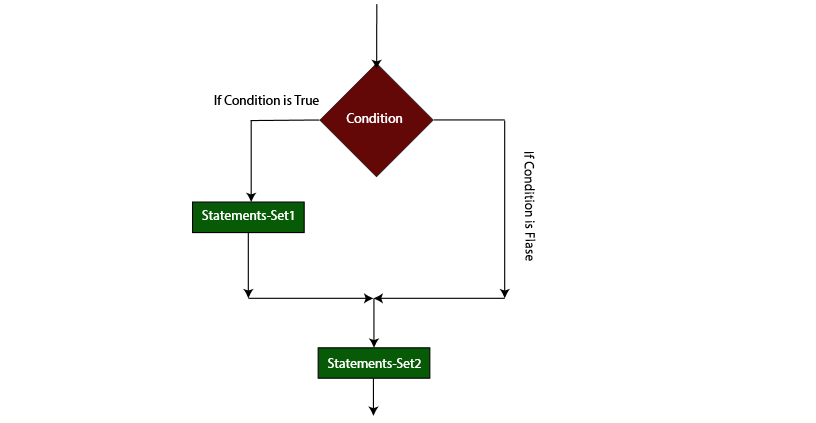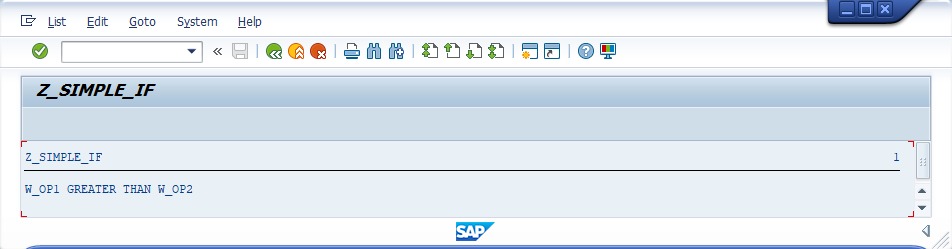###### Summary -

In this topic, we described about the below sections -

IF statement is a simple control statement that are used to verify one or more logical expressions/conditions. IF statement consists of a one or more logical expressions/Conditions followed by one or more statements.

ENDIF scope terminator is mandatory with if statement. Each statement in IF statement should ends with period(.) including IF and ENDIF.

#### Syntax -

``````IF <logical-expression>.
.
Statements-set1.
.
ENDIF.
Statement-set2.
``````

Below diagram describes the flow of IF statement -If the specified logical-expression is determined as true, then the statements-set1 executed. Next, the control goes to statements-set2. If the specified logical-expression is determined as false, then the control directly goes to statements-set2.

#### Example -

Below example shows how the IF statement coded in the application program.

#### Code -

``````*&---------------------------------------------------------------------*
*& Report  Z_SIMPLE_IF
*&---------------------------------------------------------------------*
*& Written by TutorialsCampus
*&---------------------------------------------------------------------*

REPORT  Z_SIMPLE_IF.

* Declaring Variable
DATA: W_OP1 TYPE I VALUE 90,
W_OP2 TYPE I VALUE 70.

* IF condition to verify which variable is bigger
IF W_OP1 GT W_OP2.
WRITE 'W_OP1 GREATER THAN W_OP2'.
ENDIF.
``````

#### Output -#### Explaining Example -

In the above example, each and every statement is preceeded with a comment to explain about the statement. Go through them to get clear understanding of example code.

IF W_OP1 GT W_OP2, verifying W_OP1 is GREATER THAN (GT) W_OP2. If yes, display 'W_OP1 GREATER THAN W_OP2'.